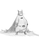111325浏览
RSI DIVERGENCE is a difference between a fast and a slow RSI . Default values are 5 for the fast one and 14 for the slow one.
You can use this indicator in 2 different ways:
• normal RSI: check double or triple top/bottom on a chart meanwhile RSI is descending/ascending (check the example on chart)
• signal line: when RSI Divergence cross zero line from bottom to top you get a buy signal (the line become green), vice versa when the RSI Divergence cross zero line in the opposite way you get a sell signal (the line become red)

## 评论@Shizaru thanks for the nice code. Looks really great.
Is it possible that the script also draws the dotted line as in your example on two or three top / bottom ?AgeM
@AgeM, @jregan11 If you need a hand with this let me know. I'm happy to code something. Just PM me.DreamsDefined
@DreamsDefined, I know its a pretty old comment but did you able to code to send an alert?jregan11
@jregan11, add this to the bottom of the script

divlong = divergence < 0 and divergence >=0
divshort = divergence > 0 and divergence <=0

data1 = divlong
plotshape(data1, style=shape.triangleup,location=location.bottom, color=green , title="DivUp")

data2 = divshort
plotshape(data2, style=shape.triangledown, location=location.top, color=red,title="DivDown")Minimal_Est
@Minimal_Est, yes definitely works. Here's the full script:

src_fast = close, len_fast = input(10, minval=1, title="Length Fast RSI")
src_slow = close, len_slow = input(33,minval=1, title="Length Slow RSI")
up_fast = rma(max(change(src_fast), 0), len_fast)
down_fast = rma(-min(change(src_fast), 0), len_fast)
rsi_fast = down_fast == 0 ? 100 : up_fast == 0 ? 0 : 100 - (100 / (1 + up_fast / down_fast))
up_slow = rma(max(change(src_slow), 0), len_slow)
down_slow = rma(-min(change(src_slow), 0), len_slow)
rsi_slow = down_slow == 0 ? 100 : up_slow == 0 ? 0 : 100 - (100 / (1 + up_slow / down_slow))
//plotfast = plot(rsi_fast, color=blue)
//plotslow = plot(rsi_slow, color=orange)
divergence = rsi_fast - rsi_slow
plotdiv = plot(divergence, color = divergence > 0 ? green:red, linewidth = 3)
//band1 = hline(70,color=green)
//band0 = hline(30,color=red)
band = hline(0)

divlong = divergence < 0 and divergence >=0
divshort = divergence > 0 and divergence <=0